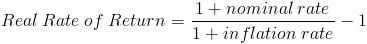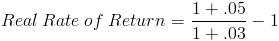# Real Rate of ReturnThe real rate of return formula is the sum of one plus the nominal rate divided by the sum of one plus the inflation rate which then is subtracted by one. The formula for the real rate of return can be used to determine the effective return on an investment after adjusting for inflation.

The nominal rate is the stated rate or normal return that is not adjusted for inflation.

The rate of inflation is calculated based on the changes in price indices which are the price on a group of goods. One of the most commonly used price indices is the consumer price index(CPI). Although the consumer price index is widely used, a company or investor may want to consider using another price index or even their own group of goods that relates more to their business when calculating the real rate of return.

For quick calculation, an individual may choose to approximate the real rate of return by using the simple formula of nominal rate - inflation rate.

## Example of Real Rate of Return Formula

An example of the real rate of return formula would be an individual who wants to determine how much goods they can buy at the end of one year after leaving their money in a money market account that earns interest.

For this example of the real rate of return formula, we must assume that the individual wants to purchase the exact same goods and same proportion of goods that the consumer price index uses considering that it is used often to measure inflation.

For this example of the real rate of return formula, the money market yield is 5%, inflation is 3%, and the starting balance is \$1000. Using the real rate of return formula, this example would showwhich would return a real rate of 1.942%. With a \$1000 starting balance, the individual could purchase \$1,019.42 of goods based on today's cost. This example of the real rate of return formula can be checked by multiplying the \$1019.42 by (1.03), the inflation rate plus one, which results in a \$1050 balance which would be the normal return on a 5% yield.

New to Finance?